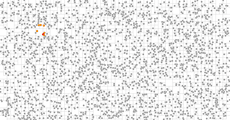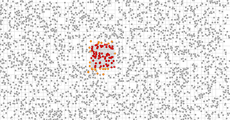# W3cubDocs

/D3.js 5

A quadtree recursively partitions two-dimensional space into squares, dividing each square into four equally-sized squares. Each distinct point exists in a unique leaf node; coincident points are represented by a linked list. Quadtrees can accelerate various spatial operations, such as the Barnes–Hut approximation for computing many-body forces, collision detection, and searching for nearby points.## Installing

If you use NPM, `npm install d3-quadtree`. Otherwise, download the latest release. You can also load directly from d3js.org, either as a standalone library or as part of D3 4.0. AMD, CommonJS, and vanilla environments are supported. In vanilla, a `d3` global is exported:

```<script src="https://d3js.org/d3-quadtree.v1.min.js"></script>
<script>

</script>```

## API Reference

Creates a new, empty quadtree with an empty extent and the default x- and y-accessors. If data is specified, adds the specified array of data to the quadtree. This is equivalent to:

```var tree = d3.quadtree()

If x and y are also specified, sets the x- and y- accessors to the specified functions before adding the specified array of data to the quadtree, equivalent to:

```var tree = d3.quadtree()
.x(x)
.y(y)

If x is specified, sets the current x-coordinate accessor and returns the quadtree. If x is not specified, returns the current x-accessor, which defaults to:

```function x(d) {
return d;
}```

The x-acccessor is used to derive the x-coordinate of data when adding to and removing from the tree. It is also used when finding to re-access the coordinates of data previously added to the tree; therefore, the x- and y-accessors must be consistent, returning the same value given the same input.

If y is specified, sets the current y-coordinate accessor and returns the quadtree. If y is not specified, returns the current y-accessor, which defaults to:

```function y(d) {
return d;
}```

The y-acccessor is used to derive the y-coordinate of data when adding to and removing from the tree. It is also used when finding to re-access the coordinates of data previously added to the tree; therefore, the x- and y-accessors must be consistent, returning the same value given the same input.

If extent is specified, expands the quadtree to cover the specified points [[x0, y0], [x1, y1]] and returns the quadtree. If extent is not specified, returns the quadtree’s current extent [[x0, y0], [x1, y1]], where x0 and y0 are the inclusive lower bounds and x1 and y1 are the inclusive upper bounds, or undefined if the quadtree has no extent. The extent may also be expanded by calling quadtree.cover or quadtree.add.

Expands the quadtree to cover the specified point ⟨x,y⟩, and returns the quadtree. If the quadtree’s extent already covers the specified point, this method does nothing. If the quadtree has an extent, the extent is repeatedly doubled to cover the specified point, wrapping the root node as necessary; if the quadtree is empty, the extent is initialized to the extent [[⌊x⌋, ⌊y⌋], [⌈x⌉, ⌈y⌉]]. (Rounding is necessary such that if the extent is later doubled, the boundaries of existing quadrants do not change due to floating point error.)

Adds the specified datum to the quadtree, deriving its coordinates ⟨x,y⟩ using the current x- and y-accessors, and returns the quadtree. If the new point is outside the current extent of the quadtree, the quadtree is automatically expanded to cover the new point.

Adds the specified array of data to the quadtree, deriving each element’s coordinates ⟨x,y⟩ using the current x- and y-accessors, and return this quadtree. This is approximately equivalent to calling quadtree.add repeatedly:

```for (var i = 0, n = data.length; i < n; ++i) {
}```

However, this method results in a more compact quadtree because the extent of the data is computed first before adding the data.

Removes the specified datum to the quadtree, deriving its coordinates ⟨x,y⟩ using the current x- and y-accessors, and returns the quadtree. If the specified datum does not exist in this quadtree, this method does nothing.

Returns a copy of the quadtree. All nodes in the returned quadtree are identical copies of the corresponding node in the quadtree; however, any data in the quadtree is shared by reference and not copied.

Returns the root node of the quadtree.

Returns an array of all data in the quadtree.

Returns the total number of data in the quadtree.

Returns the datum closest to the position ⟨x,y⟩ with the given search radius. If radius is not specified, it defaults to infinity. If there is no datum within the search area, returns undefined.

Visits each node in the quadtree in pre-order traversal, invoking the specified callback with arguments node, x0, y0, x1, y1 for each node, where node is the node being visited, ⟨x0, y0⟩ are the lower bounds of the node, and ⟨x1, y1⟩ are the upper bounds, and returns the quadtree. (Assuming that positive x is right and positive y is down, as is typically the case in Canvas and SVG, ⟨x0, y0⟩ is the top-left corner and ⟨x1, y1⟩ is the lower-right corner; however, the coordinate system is arbitrary, so more formally x0 <= x1 and y0 <= y1.)

If the callback returns true for a given node, then the children of that node are not visited; otherwise, all child nodes are visited. This can be used to quickly visit only parts of the tree, for example when using the Barnes–Hut approximation. Note, however, that child quadrants are always visited in sibling order: top-left, top-right, bottom-left, bottom-right. In cases such as search, visiting siblings in a specific order may be faster.

Visits each node in the quadtree in post-order traversal, invoking the specified callback with arguments node, x0, y0, x1, y1 for each node, where node is the node being visited, ⟨x0, y0⟩ are the lower bounds of the node, and ⟨x1, y1⟩ are the upper bounds, and returns the quadtree. (Assuming that positive x is right and positive y is down, as is typically the case in Canvas and SVG, ⟨x0, y0⟩ is the top-left corner and ⟨x1, y1⟩ is the lower-right corner; however, the coordinate system is arbitrary, so more formally x0 <= x1 and y0 <= y1.) Returns root.

### Nodes

Internal nodes of the quadtree are represented as four-element arrays in left-to-right, top-to-bottom order:

• `0` - the top-left quadrant, if any.
• `1` - the top-right quadrant, if any.
• `2` - the bottom-left quadrant, if any.
• `3` - the bottom-right quadrant, if any.

A child quadrant may be undefined if it is empty.

Leaf nodes are represented as objects with the following properties:

• `data` - the data associated with this point, as passed to quadtree.add.
• `next` - the next datum in this leaf, if any.

The `length` property may be used to distinguish leaf nodes from internal nodes: it is undefined for leaf nodes, and 4 for internal nodes. For example, to iterate over all data in a leaf node:

`if (!node.length) do console.log(node.data); while (node = node.next);`

The point’s x- and y-coordinates must not be modified while the point is in the quadtree. To update a point’s position, remove the point and then re-add it to the quadtree at the new position. Alternatively, you may discard the existing quadtree entirely and create a new one from scratch; this may be more efficient if many of the points have moved.